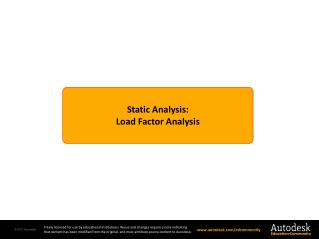DownloadDownload Presentation# Static Analysis: Load Factor Analysis

Télécharger la présentation## Static Analysis: Load Factor Analysis

- - - - - - - - - - - - - - - - - - - - - - - - - - - E N D - - - - - - - - - - - - - - - - - - - - - - - - - - -
##### Presentation Transcript

1. Static Analysis: Load Factor Analysis

2. Objectives Section II - Static Analysis Module 8 - Load Factor Analysis Page 2 The objective of this module is to introduce the equations and numerical methods used to determine if a system is stable. • Buckling is a failure mode, due to compressive components becoming unstable. • The type of analysis presented in this module is frequently called load factor, linear buckling, or eigenbuckling analysis.

3. Load Deflection Curve Section II - Static Analysis Module 8 - Load Factor Analysis Page 3 • The figure shows the force versus deflection curve of a system. • As the load is increased, the slope (tangent stiffness) progresses to a point at which it is zero. • When the stiffness is zero, the application of an infinitesimally small load will cause a very large displacement. • This type of behavior is typical of a system subjected to compressive loading. F u

4. Governing Equations Section II - Static Analysis Module 8 - Load Factor Analysis Page 4 • The governing equations for a static finite element analysis can be written as • The tangent stiffness matrix, , has three components where are the linear, displacement, and stress dependent contributions. are the displacement increments.

5. Governing Equations Section II - Static Analysis Module 8 - Load Factor Analysis Page 5 is the unbalanced load array. It is the difference between two arrays. is an array of external forces acting on the nodes. This array is obtained from the external virtual work term. is an array of node forces associated with the stresses inside the body. This array is obtained from the internal virtual work term.

6. Zero Tangent Stiffness Matrix Section II - Static Analysis Module 8 - Load Factor Analysis Page 6 • When the system is in equilibrium, the unbalanced load vector is equal to zero and the equations reduce to • There is the possibility that the system is unstable and that another equilibrium configuration exists that the system could move to without an increase in the external loads • The stress levels at which unstable configurations exist can be determined by introducing a Load Factor (l) into the above equation

7. Eigenvalue Problem Section II - Static Analysis Module 8 - Load Factor Analysis Page 7 • This equation is a homogeneous set of equations that has a solution only when the determinant of the coefficient matrix is zero. • The determinant is a polynomial that will be equal to zero only for specific values of l. The order of the polynomial is equal to the number of equations. det Characteristic Polynomial l1 l3 l l2